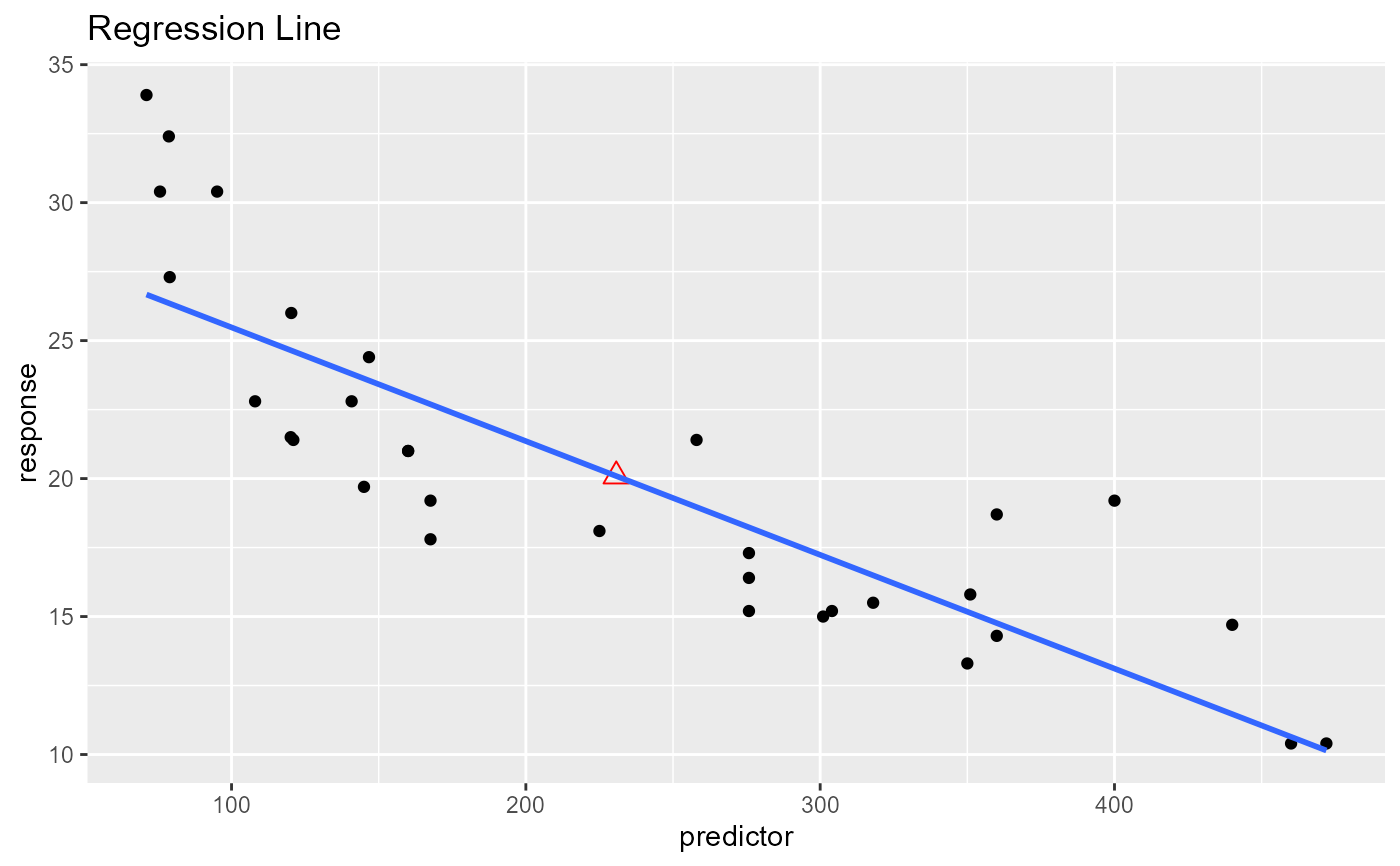Plot to demonstrate that the regression line always passes through mean of the response and predictor variables.

ols_plot_reg_line(response, predictor, print_plot = TRUE)

## Arguments

response Response variable. Predictor variable. logical; if TRUE, prints the plot else returns a plot object.

## Deprecated Function

ols_reg_line() has been deprecated. Instead use ols_plot_reg_line().

## Examples

ols_plot_reg_line(mtcars$mpg, mtcars$disp)